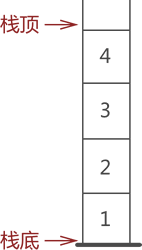# 温故：栈|数据结构

## 基本概念• 向栈中添加数据，即 `进栈``入栈``压栈`, 从栈顶进
• 从栈中取出数据，即 `出栈`，从栈顶出

• 顺序栈
• 链栈

## JDK中的实现

``````public class Stack<E> extends Vector<E> {
public Stack() {
}

public E push(E item) {

return item;
}

public synchronized E pop() {
E       obj;
int     len = size();

obj = peek();
removeElementAt(len - 1);

return obj;
}

public synchronized E peek() {
int     len = size();

if (len == 0)
throw new EmptyStackException();
return elementAt(len - 1);
}

public boolean empty() {
return size() == 0;
}

public synchronized int search(Object o) {
int i = lastIndexOf(o);

if (i >= 0) {
return size() - i;
}
return -1;
}
}
``````

## 应用场景脑暴

• 页面浏览特性
• 页面导航，面包屑导航
• 页面信息存储
• 分步求解中，中间结果的存取特性满足 `后进先出` 特性
• 进制转换
• 括号等成对匹配

## 算法题练练手

### 简单题：有效的括号

``````左括号必须用相同类型的右括号闭合。

``````

``````输入：s = "()"

``````

``````输入：s = "()[]{}"

``````

``````输入：s = "(]"

``````

``````输入：s = "([)]"

``````

``````输入：s = "{[]}"

``````

``````1 <= s.length <= 10,000 (注:原内容为10的4次方)
s 仅由括号 '()[]{}' 组成
``````

• 需要从左到右遍历整个字符串，并且匹配成对的括号
• 发现 `左括号` 时，需要先作为 `中间结果`
• 当发现 `右括号` 时，检查 `中间结果``最新` 的左括号是否对应:
• 如果不存在左括号、或者不对应，则非法
• 如果对应，则从 `中间结果` 中移除。
• 遍历完后，`中间结果` 被完全匹配，则合法。

``````static public class Q20 {
private static final Map<Character, Character> map = new HashMap<Character, Character>() {{
put('{', '}');
put('[', ']');
put('(', ')');
}};

public static boolean isValid(String s) {
final int length = s.length();

if (length % 2 == 1) //奇数一定不匹配，快速失败
return false;

final Stack<Character> stack = new Stack<>();

for (int i = 0; i < length; i++) {
Character c = s.charAt(i);
if (map.containsKey(c)) { //左括号存入栈
stack.push(c);
} else { //右括号处理
// 如果不存在左括号、或者不对应，则非法
if (stack.isEmpty() /*右边括号多余或者先于对应的左括号*/
|| c != map.get(stack.pop()) /*类型不匹配*/
)
return false;
}
}

// 遍历完后，栈中的左括号被完全匹配，则合法。
return stack.isEmpty();
}
}
``````

### 中等题： 行星碰撞

``````输入：asteroids = [5,10,-5]

``````

``````输入：asteroids = [8,-8]

``````

``````输入：asteroids = [10,2,-5]

``````

``````输入：asteroids = [-2,-1,1,2]

``````
``````提示：
2 <= asteroids.length <= 10,000 (注:原内容为10的4次方)
-1000 <= asteroids[i] <= 1000
asteroids[i] != 0
``````

• 考虑它被撞击的问题
• 考虑它撞击别人的问题

• 定义栈A，用于存储幸存的行星
• `asteroids` 按序取出行星 ast，
• `碰撞问题`：考虑 ast 与 A 中的行星可能发生的碰撞 (从栈头到栈尾)
• 如果无碰撞，则入栈
• 如果有碰撞，又分为3种可能
• ast 赢了, A栈头的行星需要出栈，继续考虑 `碰撞问题`
• ast 输了，从 `asteroids` 取下一个行星（如果不存在，则整个过程结束），继续考虑 `碰撞问题`
• 同归于尽，A栈头的行星需要出栈，从 `asteroids` 取下一个行星（如果不存在，则整个过程结束），继续考虑 `碰撞问题`

``````static public class Q735 {

public static int[] asteroidCollision(int[] asteroids) {
Stack<Integer> stack = new Stack<>();

int i = 0;
final int size = asteroids.length;
int top;

while (i < size) {

int target = asteroids[i];
//init as -1 mask it not crash
int crashResult = -1;

// + & - will crash
while (!stack.isEmpty() && stack.peek() > 0 && target < 0) {
top = stack.peek();

crashResult = top + target;
//handle crash
// crash has 3 result:
// positive: the target lost, next round
// zero: both lost, next round
// negative: left lost, if the top of current stack is positive, *if exist*, will crash
// or just push into stack

if (crashResult == 0) {
//both lost
stack.pop();
//break the loop
break;
} else if (crashResult < 0) {
//target win
stack.pop();
} else {
break;
}
}

// target win all or won't crash,push into stack
if (crashResult < 0)
stack.push(target);
i++;
}

i = stack.size();
int[] ret = new int[i];
while (i > 0) {
ret[i - 1] = stack.pop();
i--;
}

return ret;
}
}
``````

``````static public class Q735 {
//来源：力扣（LeetCode）
//链接：https://leetcode-cn.com/problems/asteroid-collision
//著作权归领扣网络所有。商业转载请联系官方授权，非商业转载请注明出处。
public static int[] asteroidCollision(int[] asteroids) {
Stack<Integer> stack = new Stack<>();
for (int ast : asteroids) {
collision:
{
while (!stack.isEmpty() && ast < 0 && 0 < stack.peek()) {
if (stack.peek() < -ast) {
stack.pop();
continue;
} else if (stack.peek() == -ast) {
stack.pop();
}
break collision;
}
stack.push(ast);
}
}

int[] ans = new int[stack.size()];
for (int t = ans.length - 1; t >= 0; --t) {
ans[t] = stack.pop();
}
return ans;
}
}
``````